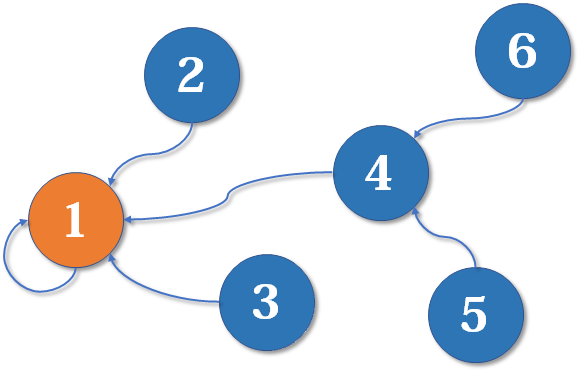• 合并（Union）：把两个不相交的集合合并为一个集合。
• 查询（Find）：查询两个元素是否在同一个集合中。

（洛谷P1551）亲戚

P行，每行一个’Yes’或’No’。表示第i个询问的答案为“具有”或“不具有”亲戚关系。

## 并查集的引入

### 初始化

int fa[MAXN];
inline void init(int n)
{
for (int i = 1; i <= n; ++i)
fa[i] = i;
}

### 查询

int find(int x)
{
if(fa[x] == x)
return x;
else
return find(fa[x]);
}

### 合并

inline void merge(int i, int j)
{
fa[find(i)] = find(j);
}

## 路径压缩

### 合并（路径压缩）

int find(int x)
{
if(x == fa[x])
return x;
else{
fa[x] = find(fa[x]);  //父节点设为根节点
return fa[x];         //返回父节点
}
}

int find(int x)
{
return x == fa[x] ? x : (fa[x] = find(fa[x]));
}

## 按秩合并

### 初始化（按秩合并）

inline void init(int n)
{
for (int i = 1; i <= n; ++i)
{
fa[i] = i;
rank[i] = 1;
}
}

### 合并（按秩合并）

inline void merge(int i, int j)
{
int x = find(i), y = find(j);    //先找到两个根节点
if (rank[x] <= rank[y])
fa[x] = y;
else
fa[y] = x;
if (rank[x] == rank[y] && x != y)
rank[y]++;                   //如果深度相同且根节点不同，则新的根节点的深度+1
}

## 并查集的应用

#include <cstdio>
#define MAXN 5005
int fa[MAXN], rank[MAXN];
inline void init(int n)
{
for (int i = 1; i <= n; ++i)
{
fa[i] = i;
rank[i] = 1;
}
}
int find(int x)
{
return x == fa[x] ? x : (fa[x] = find(fa[x]));
}
inline void merge(int i, int j)
{
int x = find(i), y = find(j);
if (rank[x] <= rank[y])
fa[x] = y;
else
fa[y] = x;
if (rank[x] == rank[y] && x != y)
rank[y]++;
}
int main()
{
int n, m, p, x, y;
scanf("%d%d%d", &n, &m, &p);
init(n);
for (int i = 0; i < m; ++i)
{
scanf("%d%d", &x, &y);
merge(x, y);
}
for (int i = 0; i < p; ++i)
{
scanf("%d%d", &x, &y);
printf("%s\n", find(x) == find(y) ? "Yes" : "No");
}
return 0;
}


（NOIP提高组2017年D2T1 洛谷P3958 奶酪）行，分别对应组数据的答案，如果在第组数据中，Jerry 能从下表面跑到上表面，则输出Yes，如果不能，则输出No（均不包含引号）。

#include <cstdio>
#include <cstring>
#define MAXN 1005
typedef long long ll;
int fa[MAXN], rank[MAXN];
ll X[MAXN], Y[MAXN], Z[MAXN];
inline bool next_to(ll x1, ll y1, ll z1, ll x2, ll y2, ll z2, ll r)
{
return (x1 - x2) * (x1 - x2) + (y1 - y2) * (y1 - y2) + (z1 - z2) * (z1 - z2) <= 4 * r * r;
//判断两个空洞是否相交或相切
}
inline void init(int n)
{
for (int i = 1; i <= n; ++i)
{
fa[i] = i;
rank[i] = 1;
}
}
int find(int x)
{
return x == fa[x] ? x : (fa[x] = find(fa[x]));
}
inline void merge(int i, int j)
{
int x = find(i), y = find(j);
if (rank[x] <= rank[y])
fa[x] = y;
else
fa[y] = x;
if (rank[x] == rank[y] && x != y)
rank[y]++;
}
int main()
{
int T, n, h;
ll r;
scanf("%d", &T);
for (int I = 0; I < T; ++I)
{
memset(X, 0, sizeof(X));
memset(Y, 0, sizeof(Y));
memset(Z, 0, sizeof(Z));
scanf("%d%d%lld", &n, &h, &r);
init(n);
fa = 1001; //用1001代表底部
fa = 1002; //用1002代表顶部
for (int i = 1; i <= n; ++i)
scanf("%lld%lld%lld", X + i, Y + i, Z + i);
for (int i = 1; i <= n; ++i)
{
if (Z[i] <= r)
merge(i, 1001); //与底部接触的空洞与底部合并
if (Z[i] + r >= h)
merge(i, 1002); //与顶部接触的空洞与顶部合并
}
for (int i = 1; i <= n; ++i)
{
for (int j = i + 1; j <= n; ++j)
{
if (next_to(X[i], Y[i], Z[i], X[j], Y[j], Z[j], r))
merge(i, j); //遍历所有空洞，合并相交或相切的球
}
}
printf("%s\n", find(1001) == find(1002) ? "Yes" : "No");
}
return 0;
}


Pecco：算法学习笔记（目录）zhuanlan.zhihu.com